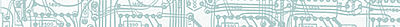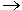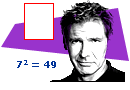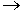# Understanding a Conditional Statement## Definition: A Conditional Statement is...

symbolized by pq, it is an if-then statement in which p is a hypothesis and q is a conclusion. The logical connector in a conditional statement is denoted by the symbol. The conditional is defined to be true unless a true hypothesis leads to a false conclusion. A truth table for pq is shown below.

 p q pq T T T T F F F T T F F T

In the truth table above, pq is only false when the hypothesis (p) is true and the conclusion (q) is false; otherwise it is true. Note that a conditional is a compound statement. Now that we have defined a conditional, we can apply it to Example 1.

Example 1:

 Given: p: I do my homework. q: I get my allowance. Problem: What does pq represent?Solution: In Example 1, the sentence, "I do my homework" is the hypothesis and the sentence, "I get my allowance" is the conclusion. Thus, the conditional pq represents the hypothetical proposition, "If I do my homework, then I get an allowance." However, as you can see from the truth table above, doing your homework does not guarantee that you will get an allowance! In other words, there is not always a cause-and-effect relationship between the hypothesis and conclusion of a conditional statement.

Example 2:

 Given: a: The sun is made of gas. b: 3 is a prime number. Problem: Write ab as a sentence. Then construct a truth table for this conditional.

Solution: The conditional ab represents "If the sun is made of gas, then 3 is a prime number."

 a b ab T T T T F F F T T F F T

In Example 2, "The sun is made of gas" is the hypothesis and "3 is a prime number" is the conclusion. Note that the logical meaning of this conditional statement is not the same as its intuitive meaning. In logic, the conditional is defined to be true unless a true hypothesis leads to a false conclusion. The implication of ab is that: since the sun is made of gas, this makes 3 a prime number. However, intuitively, we know that this is false because the sun and the number three have nothing to do with one another! Therefore, the logical conditional allows implications to be true even when the hypothesis and the conclusion have no logical connection.

Example 3:

 Given: x: Gisele has a math assignment. y: David owns a car. Problem: Write xy as a sentence.

Solution: The conditional xy represents, "If Gisele has a math assignment, then David owns a car..

In the following examples, we are given the truth values of the hypothesis and the conclusion and asked to determine the truth value of the conditional.

Example 4:

 Given: r: 8 is an odd number. false s: 9 is composite. true Problem: What is the truth value of  rs?

Solution: Since hypothesis r is false and conclusion s is true, the conditional rs is true.

Example 5:

 Given: r: 8 is an odd number. false s: 9 is composite. true Problem: What is the truth value of  sr?

Solution: Since hypothesis s is true and conclusion r is false, the conditional sr is false.

Example 6:Given: p: 72 = 49. true q: A rectangle does not have 4 sides. false r: Harrison Ford is an American actor. true s: A square is not a quadrilateral. false Problem: Write each conditional below as a sentence. Then indicate its truth value.
 1 pq If 72 is equal to 49, then a rectangle does not have 4 sides. false 2 qr If a rectangle does not have 4 sides, then Harrison Ford is an American actor. true 3 pr If 72 is equal to 49, then Harrison Ford is an American actor. true 4 qs If a rectangle does not have 4 sides, then a square is not a quadrilateral. true 5 r~p If Harrison Ford is an American actor, then 72 is not equal to 49. false 6 ~rp If Harrison Ford is not an American actor, then 72 is equal to 49. true

Note that in item 5, the conclusion is the negation of p. Also, in item 6, the hypothesis is the negation of r.

Summary: A conditional statement, symbolized by pq, is an if-then statement in which p is a hypothesis and q is a conclusion. The conditional is defined to be true unless a true hypothesis leads to a false conclusion.

### Exercises

Directions: Read each question below. Select your answer by clicking on its button. Feedback to your answer is provided in the RESULTS BOX. If you make a mistake, choose a different button.

 1. Which of the following is a conditional statement? Amy plays soccer or Bill plays hockey.Bill plays hockey when Amy plays soccer.If Amy plays soccer then Bill plays hockey.None of the above. RESULTS BOX:
2.
 Given: r: You give me twenty dollars. s: I will be your best friend. Problem: Which of the following statements represents, "If you give me twenty dollars, then I will be your best friend"?

rs
rs
sr
None of the above.

RESULTS BOX:

 3. What is the truth value of rs when the hypothesis is false and the conclusion is true in Example 2? TrueFalseNot enough information was given.None of the above. RESULTS BOX:
4.
 Given: a: x is prime. b: x is odd. Problem: What is the truth value of ab when x = 2?

True
False
Not enough information was given.
None of the above.

RESULTS BOX:

 5. What is the truth value of ab when x = 9 in Exercise 4? TrueFalseNot enough information was given.None of the above. RESULTS BOX:
 Lessons on Symbolic Logic Negation Conjunction Disjunction Conditional Compound Biconditional Tautologies Equivalence Practice Exercises Challenge Exercises Solutions